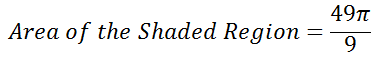## Tuesday, October 7, 2014

### A step-back SAT/GRE problem -- Circles

I've been thinking a lot about video learning, particularly video-assisted self-study. One of the ideas I've come up with is the step-back problem.

Sometimes, when you're trying to teach yourself a subject or study for a test like the SAT or GRE, you will run into a problem that still confuses you after you've gone through the explanation. If you have access to a live instructor,  you can always ask for a more detailed explanation but what do you do if you are trying to learn the subject from YouTube videos?

My thought is to pair up problems of medium to high difficulty with problems that use some of the same concepts but are much simpler.

Here's an example. The following is very similar to some problems you are likely to see on the SAT or GRE. Try it on your own then check below the fold for the answer. If you didn't get the right answer and still don't understand what you got wrong even after reading the explanation, try the second problem.

Circle 1

The radius of circle 1 is 5. Both line segments pass through the center of the circle. Find the area of the shaded region.

Explanation:

There are two parts to solving this -- finding out the area of the circle and figuring out how much of the circle is shaded -- and it doesn't matter which we do first. Both steps are fairly simple once you see where you're going but figuring out the portion shaded might be somewhat less obvious so let's start there.

Think about the relationship between 5x and x.

You probably remember that the measure of a straight angle is one hundred and eighty degrees so we know the sum of these two angles and should be able to find x.

Since there are 360 degrees in a circle, 30 degrees represent one 12th of a circle and since the angle of the shaded region is also x degrees (because opposite angles are congruent), the area of the shaded region is one 12th the area of the circle.

Finding the area of Circle 1 is straightforward if you remember the formula for the area of a circle.

Put those two together and you get:

Still confused, try this problem

Circle 2

The radius of Circle 2 is 7. Find the area of the shaded region.

Explanation:

We need to figure out how big the circle is and how much of it is being shaded.

We can get the first from the formula for the area of a circle:

And since there are 360 degrees in a circle, a forty degree segment is one 9th of the circle.

Put these two together to get